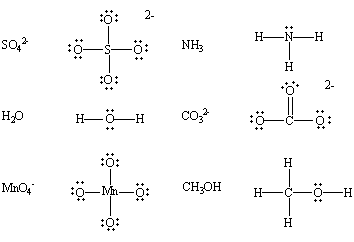# Acid and Base

Table of Content:

Robert Boyle defined:

a) Acid as having,

1) sour taste 2) corrosive and 3) change the colour of certain vegetable dyes, such as litmus.

b) Base as 1) slippery and 2) change the colour of litmus from red to blue.

Based on their occurrence, they are divided into two types- Natural and mineral acids.

Natural Acids: These are obtained from natural sources, such as fruits and animal products.

e.g. lactic, citric, and tartaric acid etc.

Mineral Acids: Mineral acids are acids prepared from minerals.

Example, Hydrochloric acid (HCl), Sulphuric Acid (H2SO4), and nitric acid (HNO3) etc.

## Arrhenius Definition of Acids and Bases

Arrhenius suggested that-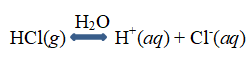a) Acid is a substance that ionizes in water to give H+ ions.

Hydrogen chloride ionizes in water to give hydrogen (H+).

Depending on the number of a proton the acid gives, it can be classified as mono, di, tribasic acid.

Monobasic acids: HCl, Nitric acid, acetic acid

Dibasic acids: Sulphuric acid, phosphorous acid

Tribasic acids: phosphoric acid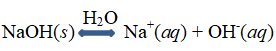b) The base is a substance that ionize in water to give OH ions.

NaOH dissociates in water to give the hydroxide (OH)

Based on the number of available hydroxide ion, a base can be mono, di, tri acidic bases.

Mono basic: NaOH, NH4OH

Dibasic: Ca(OH)2, Zn(OH)2

Tribasic: Fe(OH)3, Al(OH)3

This theory explains the similarity of properties among acids and bases neutralization of bases by acid and vice versa. Acids provide the H+ ion; bases provide the OH ion; these ions combine to form water.

H+(aq) + OH(aqH2O(l)

Acid and bases that ionize completely giving larger hydrogen or hydroxide ions in solutions are called strong acids and bases. HCl, HNO3, H2SO4, HCIO4, etc., are examples of strong acids and NaOH, KOH, (CH3)4NOH are strong bases.

Acids and bases that are dissociated to a limited extent giving a lesser amount of hydrogen or hydroxide ions in solutions are termed weak acids and bases.

Compounds containing ionizable hydrogen or hydroxide ions can be an acid or base. CH4 is not an acid. Similarly, CH3OH, C2H5OH, etc., having OH groups are not bases.

Disadvantages of Arrhenius theory of acid and base:

i) Applicable to aqueous solutions only as acids and bases are defined from ionization in water.

ii) Cannot explain the acidic nature of nonmetal oxides, aluminium chloride and basic nature of

ammonia, sodium carbonate, metal oxides.

## Relative Strength of Acids and Bases

All strong acid and bases are equally ionized and water is amphoteric. So they have the same acidic or basic strength in water. This is known as the leveling effect.

The strength of acids also depends upon the solvent. Acetic acid is not receptive to take up protons and has to be forced. Thus acids HCIO4, H2S04, HCl and HN03 which have nearly the same strength in water follow the order of HClO4> H2SO> HCl > HNO3 in acetic acid.

The real strength of acids can be judged by solvents. On the basis of proton interaction, solvents can be classified into four types:

• Protophilic solvents:  Solvents which have a greater tendency to accept protons, i.e., water, alcohol, liquid ammonia, etc.
• Protogenic solvents:  Solvents which have the tendency to produce protons, i.e., water, liquid hydrogen chloride, glacial acetic acid, etc.
• Amphiprotic solvents:  Solvents which act both as protophilic or protogenic, e.g., water, ammonia, ethyl alcohol, etc.
• Aprotic solvents:  Solvents which neither donate nor accept protons, e.g., benzene, carbon tetrachloride, carbon disulphide, etc.

HCI acts as an acid in the water, stronger acid in NH3, weak acid in CH3COOH, neutral in C6H6 and a weak base in HF.

## Bronsted-Lowry Concept Of Acid And Base

Bronsted acids are hydrogen-ion or proton donors.

Bronsted bases are hydrogen-ion or proton acceptors.

HCl donates a H+ ion to a water molecule to form H3O+ .

$HCl(g)+H_2O(l)\rightleftharpoons H_3O(aq)+Cl^{-}(aq)$

HCl acts as an acid and water is a base.

Neutralization, as per Bronsted model, involve transfer of an H+ ion from an acid to a base. Acids can be

i) neutral molecules. $HCl(g)+NH_3(aq)\rightleftharpoons NH_4(aq)+Cl^{-}(aq)$

ii) positive ions $NH_{4}^{+}(aq)+OH(aq)\rightleftharpoons NH_3(aq)+H_2O^{-}(l)$

iii) negative ions. $H_{2}PO_{4}^{-}(aq)+H_2O(l)\rightleftharpoons HPO_{4}^{2-}(aq)+H_3O^{-}(aq)$

## Conjugate Acid – Base Pairs

In the reaction– $HCl(aq)+NH_3(aq)\rightleftharpoons NH_{4}^{+}(aq)+H_3O^{-}(aq)$

HCl and Cldiffer by a proton and so are the NH3 and NH4+

NH4+ like HCl can donate a proton and hence an acid. Since it has come from the base ammonia, it is called as a conjugate acid of base ammonia.

Similarly, Cl like ammonia can accept a proton and hence a base. It is considered as the conjugate base of the acid HCl.

The Bronsted – Lowry transfer can be written as-

$HCl(aq)+NH_3(aq)\rightleftharpoons NH_{4}^{+}(aq)+Cl^{-}(aq)$

Or as Acid + Base → Conjugate acid + Conjugate base

Conjugate pairs

A conjugate acid contains one more H atom and +charge than the base that formed it.

A conjugate base contains one less H atom and one more negative charge than the acid-forming it.

The conjugates will always be listed on the product side of the reaction.

Strong acid forms a weak conjugate base and vice versa.

Reactions always go from strong acid/base to weak acid/base.

## Acid-Base Nature Of Compounds

1. Compounds that contain hydrogen(+1) bound to a nonmetal are called nonmetal hydrides are acidic.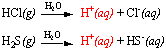2. Metal hydrides contain hydrogen (-1) bound to a metal. They give the H (or hydride) ion.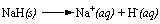The H ion, with its pair of valence electrons, can abstract an H+ ion from a water molecule.

and increase, OH ion concentration. So, in a solution, metal hydrides are bases.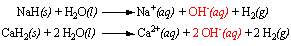3. Nonmetal oxides dissolve in water to form acids. CO2 dissolves in water to give carbonic acid,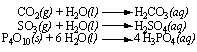4. Metal oxides, containing O2- ion, reacts with water to give a pair of OH ions and base.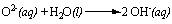Metal oxides, therefore, fit the operational definition of a base.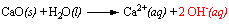5. Metal hydroxides, such as LiOH, NaOH, KOH, and Ca(OH)2, are bases.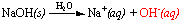The difference between the electronegativities of sodium and oxygen is very large (EN = 2.5). As a result, the electrons in the NaO bond are not shared equally, these electrons are drawn toward the more electronegative oxygen atom. NaOH, therefore, dissociates to give Na+ and OH ions when it dissolves in water.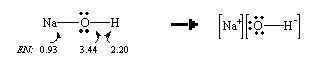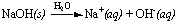6. Nonmetal hydroxides, like hypochlorous acid (HOCl), HONO2, O2S(OH)2, OP(OH)3 are

acids.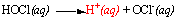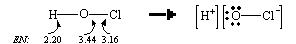Here, the difference in electronegativities of the chlorine and oxygen atoms is small (EN = 0.28). So, the electrons in the ClO bond are shared more or less equally by the two atoms. The OH bond, on the other hand, is polar (EN = 1.24)the electrons in this bond are drawn toward the more electronegative oxygen atom to form OCl and H+ ions are formed.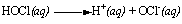The acidic hydrogen atoms in the non-metal hydroxides aren’t bound to the nitrogen, sulfur, or phosphorus atoms but only to the oxygen atom. These compounds are oxyacids.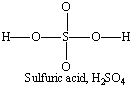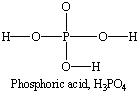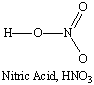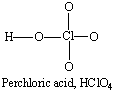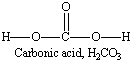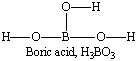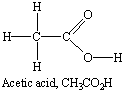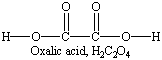7. Oxides and hydroxides that lie between the metal and nonmetal oxides, or metal and nonmetal hydroxides, are amphoteric. These compounds, such as Al2O3 and Al(OH)3, can act as either acids or bases. Al(OH)3, for example, acts as an acid when it reacts with a base.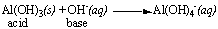Conversely, it acts as a base when it reacts with an acid.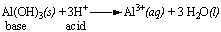## Lewis ( Electron) Concept Of Acid- Bases

Species that can accept electron pairs are acids and called as Lewis acids.

1. Molecules having a central atom with an incomplete octet (less than 8 electrons): BF3, BCl3, AlCl3, MgCl2. BeCl2. etc.
2. Molecules having a central atom with empty d-orbitals: SiX4, GeX4, TiCl4, SnX4, PX3, PF5, SF4, SeF4, TeCl4, etc.,
3. Molecules having multiple bonds between atoms of dissimilar electronegativity: CO2, SO2 and SO3. Under the influence of attacking Lewis base, one -electron pair will be shifted towards the more negative atom.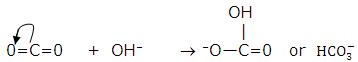4. Simple cations: Cations that have a greater tendency to accept electrons. H+, Ag+,

The following species can act as Lewis bases.

1. Neutral species having at least one lone pair of electrons: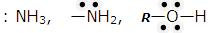2. Negatively charged species or anions: For example, chloride, cyanide, hydroxide ions, etc.,
3. It may be noted that all Bronsted bases are also Lewis bases but all Bronsted acids are not Lewis acids.

The following compounds, for example, contain nonbonding pairs of electrons.